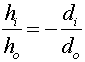Examples for lenses

Example #1

Problem:

a.) A converging lens (concave) has a focal length of 14 cm. Looking through the lens, one sees an image 20 cm behind the lens. Where is the object?

Solution:

Since the image is behind the lens, it is virtual and the distance di is negative. Using the formula,, one can solve for do.

do = 8.24 cm

b.) If the height of the object is 1.5 cm, what is the height of the image.

Solution:

Using the formula,to get

hi = 3.64 cm, upright

Example #2

Problem:

A real image of a coin is observed 34 cm beyond a lens. The image height is 1.4 cm and it is known that the actual coin is 0.7 cm high. What is the focal length of the lens?

Solution:

First, find the object distance using. Remember that since the image is real, that the image height is negative. ( do = 17 cm ). One can then useto find the focal length.

do = 11.33 cm

Lenses' index